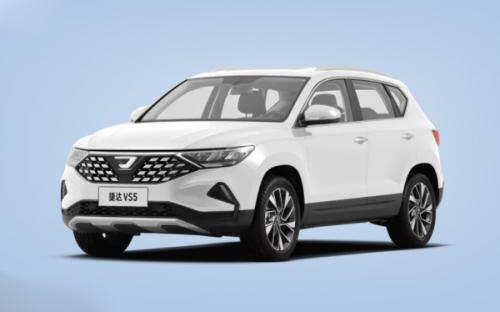## 捷达 捷达VS52019款最低售价：8.48 万元起

4419(mm)1841(mm)1616(mm)##### 配置亮点：
• 胎压监测装置

• ISOFIX儿童座椅接口

• 车身稳定控制(ESC/ESP/DSC等)

• 电动天窗

• 定速巡航

• 后倒车雷达

• 真皮座椅

• GPS导航系统

• 氙气大灯

• 后视镜加热

2019款 2019款 280TSI 自动荣耀型 (199张)
• 2019款 2019款 280TSI 自动荣耀型 (199张)
• 2019款 2019款 280TSI 手动进取型 (138张)捷达 捷达VS5 在售车型

排量 车型 厂商指导价 本地最低报价 购车工具
1.4T 2019款 280TSI 手动进取型 5挡手动 8.48万 8.48万

询底价+对比
2019款 280TSI 自动进取型 6挡手自一体 9.58万 询底价+对比
2019款 280TSI 手动悦享型 5挡手动 9.18万 9.18万

询底价+对比
2019款 280TSI 自动悦享型 6挡手自一体 10.28万 询底价+对比
2019款 280TSI 自动荣耀型 6挡手自一体 11.28万 11.28万

询底价+对比

捷达 捷达VS5 经销商

查看更多 >>
更多 >>

本地降价排名

### 捷达 捷达VS5 动力加速

捷达VS5 0-100公里加速时间分布在 0.0-秒 属于 超跑级

动力级别 加速时间 车型

捷达 捷达VS5 视频

捷达 捷达VS5 新闻资讯

# 新H6 Coupe竟全面压制捷达VS5?

试驾评测 超过12264次关注

有一位朋友来向小编问询,问我新哈弗H6Coupe智联版(以下简称新H6Coupe)和捷达VS5,价格相近的两款SUV,到底选哪一款好。

# 中国老炮与德系新品的对决

试驾评测 超过14274次关注

中国老炮与德系新品之间的对决。我们来看看来自一汽大众的“新人”捷达VS5与来自哈弗品牌的“老炮”新哈弗H6Coupe智联版(简称:新H6Coupe)的较量,看谁能在市场红海中独占鳌头。

# 新H6 Coupe对话捷达VS5:切莫班门弄斧!

试驾评测 超过14024次关注

今天,我们来看看来自一汽大众的“新人”捷达VS5与来自哈弗品牌的“老炮”新哈弗H6Coupe智联版(简称:新H6Coupe)的较量,看谁能在市场红海中独占鳌头。

# 捷达VS5想要追上新哈弗H6 Coupe智联版还需努力

试驾评测 超过14476次关注

独立品牌捷达，顶着大众光环的主推车型捷达VS5更是风头不小，然而,它真的强吗?能撼动哈弗H6Coupe智联版数月来的销冠宝座吗？我看有点难。

# 捷达VS5想要追上新哈弗H6 Coupe智联版

汽车导购 超过14042次关注

捷达VS5顶着大众光环风头不小！但SUV市场的销冠大佬依旧是新哈弗H6Coupe智联版，而新哈弗H6Coupe智联版(以下简称新H6Coupe)市场肯定度也颇高。所以，捷达VS5具备赶超它的实力吗?

# 9月售22万辆 一汽-大众三大品牌车市旺季发力

新闻 超过10599次关注

上市就热销，捷达品牌真香！捷达品牌9月销量为1.1万辆，相比既定销量目标完成度为130%。，捷达旗下两款全新车型均于9月上市，捷达VS5上市于9月5日的成都车展，捷达V...

# 捷达VS5共5款车型 成都车展上市

新闻 超过10154次关注

我们从经销商处获悉，一汽-大众捷达VS5将推出5款车型，新车预售价区间为8.98-11.98万元，将在9月5日开幕的成都车展期间上市。

# 哈弗H4乐享版 品质赶超捷达VS5

试驾评测 超过14625次关注

一直以来，合资品牌与自主品牌在SUV市场可谓“厮杀”激烈，而在年轻人逐渐成为消费主体的当下，很多自主品牌通过推陈出新，已逐渐从合资品牌中夺过市场主导权。哈弗...

猜你喜欢

﻿
• 快速找车
• 选择品牌
• 选择品牌
• A  奥迪
• A  阿斯顿·马丁
• A  阿尔法·罗密欧
• B  宝沃
• B  布加迪
• B  巴博斯
• B  保时捷
• B  宾利
• B  奔驰
• B  宝马
• B  本田
• B  别克
• B  标致
• B  比亚迪
• B  宝骏
• B  北汽制造
• B  北汽新能源
• B  北汽幻速
• B  北汽威旺
• B  北京汽车
• B  奔腾
• B  北汽绅宝
• C  长安
• C  长安商用
• C  长城
• C  昌河
• D  大众
• D  道奇
• D  DS
• D  东南
• D  东风风神
• D  东风风行
• D  东风小康
• D  东风风度
• D  东风
• F  福特
• F  丰田
• F  菲亚特
• F  法拉利
• F  福田
• F  福迪
• F  福汽启腾
• G  观致
• G  广汽传祺
• G  广汽吉奥
• G  GMC
• H  红旗
• H  汉腾汽车
• H  哈弗
• H  哈飞
• H  海格
• H  海马
• H  华颂
• H  黄海
• H  华泰
• H  恒天
• J  吉利汽车
• J  捷豹
• J  Jeep
• J  江淮
• J  江铃
• J  金杯
• J  九龙
• J  金旅
• K  凯翼
• K  凯迪拉克
• K  克莱斯勒
• K  科尼塞克
• K  卡威
• K  开瑞
• L  路虎
• L  林肯
• L  劳斯莱斯
• L  兰博基尼
• L  雷克萨斯
• L  铃木
• L  雷诺
• L  理念
• L  力帆
• L  莲花汽车
• L  猎豹
• L  路特斯
• L  陆风
• M  马自达
• M  MG
• M  MINI
• M  玛莎拉蒂
• M  摩根
• M  迈凯轮
• N  纳智捷
• O  欧宝
• O  讴歌
• O  欧朗
• Q  奇瑞
• Q  起亚
• Q  启辰
• R  日产
• R  荣威
• R  瑞麒
• S  三菱
• S  斯威汽车
• S  萨博
• S  smart
• S  斯柯达
• S  斯巴鲁
• S  思铭
• S  双龙
• S  上汽大通
• S  双环
• T  特斯拉
• T  腾势
• W  沃尔沃
• W  五菱汽车
• W  五十铃
• W  威兹曼
• W  威麟
• X  现代
• X  雪佛兰
• X  雪铁龙
• X  西雅特
• Y  一汽
• Y  英菲尼迪
• Y  英致
• Y  依维柯
• Y  野马汽车
• Y  永源
• Z  众泰
• Z  中华
• Z  中兴
• Z  知豆
• 选择车系
• 选择车系
• 车型对比
• 选择品牌
• 选择品牌
• A  奥迪
• A  阿斯顿·马丁
• A  阿尔法·罗密欧
• B  宝沃
• B  布加迪
• B  巴博斯
• B  保时捷
• B  宾利
• B  奔驰
• B  宝马
• B  本田
• B  别克
• B  标致
• B  比亚迪
• B  宝骏
• B  北汽制造
• B  北汽新能源
• B  北汽幻速
• B  北汽威旺
• B  北京汽车
• B  奔腾
• B  北汽绅宝
• C  长安
• C  长安商用
• C  长城
• C  昌河
• D  大众
• D  道奇
• D  DS
• D  东南
• D  东风风神
• D  东风风行
• D  东风小康
• D  东风风度
• D  东风
• F  福特
• F  丰田
• F  菲亚特
• F  法拉利
• F  福田
• F  福迪
• F  福汽启腾
• G  观致
• G  广汽传祺
• G  广汽吉奥
• G  GMC
• H  红旗
• H  汉腾汽车
• H  哈弗
• H  哈飞
• H  海格
• H  海马
• H  华颂
• H  黄海
• H  华泰
• H  恒天
• J  吉利汽车
• J  捷豹
• J  Jeep
• J  江淮
• J  江铃
• J  金杯
• J  九龙
• J  金旅
• K  凯翼
• K  凯迪拉克
• K  克莱斯勒
• K  科尼塞克
• K  卡威
• K  开瑞
• L  路虎
• L  林肯
• L  劳斯莱斯
• L  兰博基尼
• L  雷克萨斯
• L  铃木
• L  雷诺
• L  理念
• L  力帆
• L  莲花汽车
• L  猎豹
• L  路特斯
• L  陆风
• M  马自达
• M  MG
• M  MINI
• M  玛莎拉蒂
• M  摩根
• M  迈凯轮
• N  纳智捷
• O  欧宝
• O  讴歌
• O  欧朗
• Q  奇瑞
• Q  起亚
• Q  启辰
• R  日产
• R  荣威
• R  瑞麒
• S  三菱
• S  斯威汽车
• S  萨博
• S  smart
• S  斯柯达
• S  斯巴鲁
• S  思铭
• S  双龙
• S  上汽大通
• S  双环
• T  特斯拉
• T  腾势
• W  沃尔沃
• W  五菱汽车
• W  五十铃
• W  威兹曼
• W  威麟
• X  现代
• X  雪佛兰
• X  雪铁龙
• X  西雅特
• Y  一汽
• Y  英菲尼迪
• Y  英致
• Y  依维柯
• Y  野马汽车
• Y  永源
• Z  众泰
• Z  中华
• Z  中兴
• Z  知豆
• 选择车系
• 选择车系
• 选择车型
• 选择车型
• 意见反馈